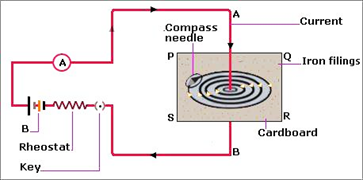### Which of the statement given below correctly describes the magnetic field near a long, straight current-carrying conductor? a. The magnetic lines of force are in a plane, perpendicular to the conductor in the form of straight lines.

Question 12.

Which of the statement given below correctly describes the magnetic field near a long, straight current-carrying conductor?

a. The magnetic lines of force are in a plane, perpendicular to the conductor in the form of straight lines.

b. The magnetic lines of force are parallel to the conductor on all the sides of the conductor.

c. The magnetic lines of force are perpendicular to the conductor going radially outward.

d. The magnetic lines of force are in concentric circles with the wire as the center, in a plane perpendicular to the conductor.

Answer:

d

When current is passed through a long, straight current-carrying wire a magnetic field is developed where magnetic field lines are in form of concentric circles around the wire in the center with their plane perpendicular to the straight wire. We can obtain these magnetic lines of force by sprinkling some iron fillings in a cardboard with a straight long wire passing through the center of the cardboard. This arrangement is connected to battery, key, rheostat, and ammeter. When current will pass through this iron fillings will get arranged in form of concentric circles around the wire.